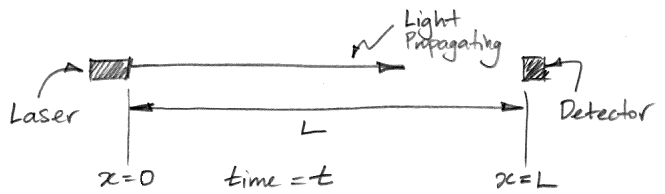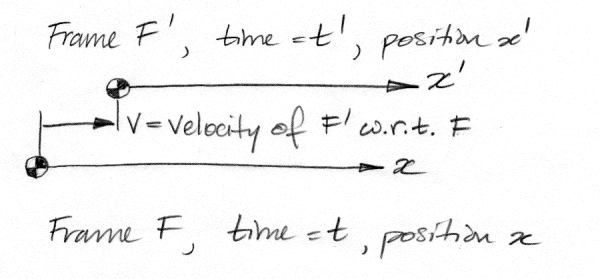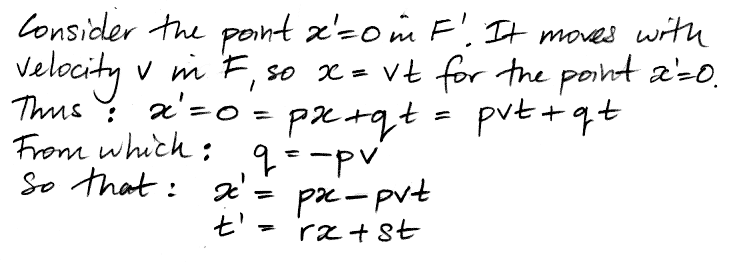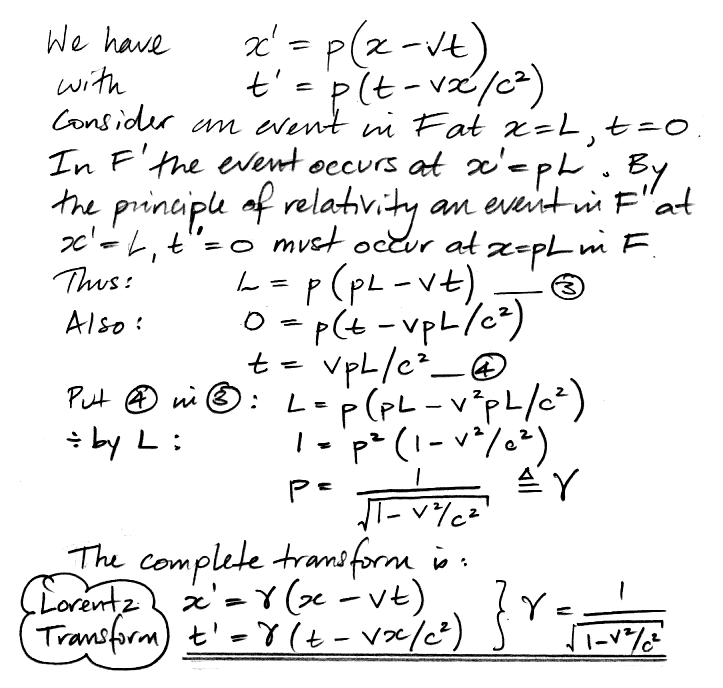# Derivation of the Lorentz Transform

© 2016-2017 Kevan Hashemi, Brandeis University

## Contents

Speed of Light
Inertial Frames
The Principle of Relativity
Transformation Function
Relative Velocity
Light Speed
Length Contraction
The Inverse Transform
Time Dilation and Length Contraction
Questions

## Introduction

Here we derive the Lorentz Transform, which is the mathematical expression of Special Relativity. The Lorentz Transform allows us to calculate how much shorter a moving ruler will appear to us as it moves by, how much slower a moving clock will run compared to a stationary clock, and how much longer an unstable sub-atomic particle will last if it is moving close to the speed of light. Our derivation will require only simple algebra to complete. We continue our investigation of fast-moving objects in Relativistic Collisions.

## Speed of Light

We have the following apparatus for measuring the speed of light. It contains a clock that measures time, t, and a ruler that measures position, x. A laser at position x = 0 m turns on at time t = 0 s. Its light propagates towards a target at position x = L. The light arrives at the detector at time t = a. The speed of light is c = L/a.Figure: Apparatus for Measuring the Speed of Light.

We set up the apparatus in our laboratory on the surface of the Earth. We point the laser in the direction of the Earth's 30 km/s orbital velocity. We measure c = 299,792 km/s. We rotate the apparatus and point the laser in the opposite direction. Some of us are expecting to get an answer 60 km/s higher this time, because the Earth is carrying the target towards the advancing light, while it carried the target away from the advancing light in the first measurement. But we measure c = 299,792 km/s again. We repeat our measurement in many orientations and we always get the same answer. We repeat our measurement in the same orientation a hundred times throughout the day, and we always get the same answer.

What we have described is a simplified version of the series of experiments performed by Michelson and Morley in 1887, and repeated in a variety of ways in the decades that followed. The speed of light is the same for all observers moving at constant velocity, regardless of how fast they move, the direction of the light, or which observer holds the source of the light.

In the following sections, we will determine how it is possible for different observers moving at different velocities to measure the same beam of light to be moving at the same speed. For brevity and clarity, but without loss of generality, we will conduct our investigation in one spatial dimension. We will arrive at the one-dimensional Lorentz Transformation, which is easily extended to three dimensions.

## Inertial Frames

For our purposes, an inertial frame of reference is a ruler that is not accelerating, accompanied by a set of synchronized clocks. The ruler measures position, x, along a line. In one direction, x increases and in the other it decreases. Along the ruler we have the clocks. Observers who are stationary with respect to the ruler agree that the clocks read exactly the same time. We say the clocks are synchronized within the frame of reference. We can check the synchronisation of the clocks at any time with a machine that travels along our ruler at a constant velocity u. We release this machine from x = 0 m at time t = b s. When it passes a clock at position x = L, the clock should read b + L/u. If the clock is wrong, we correct it.

The following diagram shows two frames of reference, F and F |. We could place them right on top of one another, so that their rulers were along the same line, but this would be hard to draw. Let us imagine that their rulers are parallel and close together. The frames are moving with respect to one another. So far as frame F is concerned, frame F | is moving at velocity v, where v is positive. Conversely, so far as frame F | is concerned, frame F is moving at −v.Figure: Two Frames of Reference Moving With Respect to One Another.

Suppose something happens in the space between the two rulers, so that observers on both rulers have a clear view of the event. This event could be a beam of light being emitted by a laser or a beam of light striking a target. When this event occurs, it does so at a position x in F and x | in F |. We make sure there is a clock right there on both rulers. The clock in F is synchronized with all other clocks in F and reads time t, while the clock in F | is synchronized with all other clocks in F | and reads time t |.

The values of t and t | could be different because one clock is far behind the other. But let us suppose, without loss of generality, that at t = 0 s and x = 0 m in F corresponds to t | = 0 s and x | = 0 in F |. At time zero in both frames, the zero-positions on the rulers of both frames are next to one another.

We are inclined to assume that the clocks facing one another across the small space between the rulers will always read the same time. And we are inclined to assume that the 1-mm divisions on the two rulers will have the same length. But we will make neither assumption. We will assume only that one frame is moving at v with respect to the other and the speed of light will be c in both frames. These two observations are so constraining that they already dictate what we will see on either side of the small gap between the rulers, so we cannot make any further assumptions. All we can do is figure out the consequences of the assumptions we have already made.

## The Principle of Relativity

The principle of relativity states that a distance or a length of time we measure will not depend upon where we place the point x = 0 m, nor the moment we choose to call t = 0 s, nor will it matter which of two frames we choose to call F or F |. We might protest that F | moves in F at v while F moves in F | at −v. But we could just as easily construct F | with x | pointing in the opposite direction, in which case each frame would be moving in the other at v, so this difference in sign cannot not break the physical symmetry between the two frames, nor cause a violation of the principle of relativity.

## Transformation Function

Consider an event that occurs at position x and time t in frame F. In frame F | the same event has position x | and time t |. Because x and t correspond uniquely to x | and t |, there exist two mathematical equations that allow us to calculate x | and t | from the values of x and t. Together, these two equations constitute the transformation function from F to F |. Our task is to determine these equations. Our first step is to argue that the transformation has the following form, where p, q, r, and s are numbers.

x | = px + qt
t | = rx + st

Suppose we keep t constant. The slope of a graph of x | versus x will be a straight line with slope p. The graphs of x | versus t for constant x, and of t | versus x for constant t, and of t | versus t for constant x, will also be straight lines. We say the transformation function is linear in x and t.

Suppose the transformation function were not linear. Suppose the slope of the graph of x | versus x for fixed t were a curved line. Without loss of generality, suppose the slope of the graph is 1.0 at x = 0 m and 2.0 at x = 1000 m. Observer A stands in F at x = 0 m and looks at the ruler in F | that moves by at velocity v. She observes that the 1-mm divisions on the moving ruler are the same length as the 1-mm divisions on her own ruler. Observer B stands in F at x = 1000 m. He looks across at the moving ruler and observes that its 1-mm divisions are half as long as the 1-mm divisions on his own ruler. Now suppose we press a button and all the distance marks on the ruler in F drop by 1000 m. Observer B is now at position x = 0 m. Our non-linear transformation tells us that B will now observe that the 1-mm markings on the moving ruler are the same size as the 1-mm markings on his own ruler. We have arrived at a contradiction: it is impossible for our choice of where to place x = 0 m on our own ruler to have any effect upon the apparent size of the 1-mm divisions of the ruler in F |.

We can use the same argument for any curvature in the graph of x | versus x for constant t, however slight. No such curvature is possible. The graph must be straight. Our argument is an application of the principle of relativity: the location of x = 0 m and the moment of t = 0 s cannot change our observations of length or duration. There is nothing impossible about the ruler appearing to shrink from the point of view of observers on F, but it is impossible for the ruler to appear to shrink by different amounts at different places or times in F. By the same argument, we can show that the graph of x | versus t for constant x must be straight, as well as the graph of t | versus x for constant t, and the graph of t | versus t for constant x.

The transformation function is linear. Our job is to deduce the constants p, q, r, and s using the following constraints: the transform will obey the principle of relativity, the transform will predict that light will travel at speed c in both frames, and the transform will agree that one frame is moving at velocity v with respect to the other.

## Relative Velocity

The point x | = 0 m moves with velocity v with respect to F. Without loss of generality, we assumed the points x | = 0 and x = 0 m were coincident at time t = 0 s in F and t | = 0 s in F |. These two constraints permit us to derive a relation between the constants p and q in our transformation function.We are sitting on a train looking outside. We are F | and the world outside is F. Houses appear to have the same dimensions they would if we were standing on the ground, but they are moving past us. For all practical purposes, we have p = 1 so that x | = xvt. At t = 0 s the two rulers are lined up and their divisions are the same length. When v << c, we expect p = 1. By the same argument, s = 1 and r = 0 s/m for v << c, so that we will obtain the familiar relation t | = t. Only when v becomes significant with respect to the speed of light will we see p ≠ 1, s ≠ 1, and r ≠ 0.

## Light Speed

Observers in both F and F | will measure the speed of a beam of light to be c. If the light propagates in the positive direction, both observers will measure it to be moving at velocity c. Suppose a beam of light leaves the point x = 0 m at time t = 0 m. At time t, an observer in F will find the beam has propagated to x = ct. At time t |, an observer in F | will find the beam has propagated to x | = ct |. The same is true for a beam of light propagating in the negative direction. Observers in F and F | will measure the beam's velocity to be −c. These two considerations, of light going in both directions, allow us to express both r and s in terms of p.At time t = 0 s, t | = −pvx/c2. For x > 0 m in F at time t = 0 s, the clocks on F | lag behind the clocks on F. The farther we go from x = 0 m, the greater the lag becomes. In the negative direction, the opposite is true: the clocks on F | are increasingly ahead of the clocks on F. Suppose two events occur simultaneously in F at time t = 0 s, but one event occurs at x = 0 m and the other occurs at x > 0 m. An observer in F |, however, will claim that the event at x > 0 m occurred before the event at x = 0 m. Events in different locations that are simultaneous in F will not be simultaneous in F |. Our transformation function preserves the speed of light, but it does not preserve simultaneity.

## Length Contraction

Consider the segment of the ruler in F that extends from x = 0 m to x = L. At time t = 0 s our transformation function tells us that one end of this segment is at x | = 0 m and the other is at x | = pL. For v << c we have p ≈ 1, but for larger v we expect p ≠ 1. We don't yet know if p < 1 or p > 1, but let us suppose p > 1. To the observer in F, the ruler in F | appears to have contracted by a factor of p.

When we measure the length of a moving object, we mark the position of its front end and its back end on our ruler. We must make these marks simultaneously, or else the object will move between the time we make the first mark and the time we make the second mark. But simultaneity is not conserved between frames. If simultaneity is not conserved, nor will length be conserved.

If the ruler in F | appears contracted by a factor of p for an observer in F, how does the ruler in F appear to an observer in F |? The principle of relativity dictates that the ruler in F will also appear to be contracted. If x = L and t = 0 s corresponds to x | = pL, then x | = L and t | = 0 s will correspond to x = pL. This length constraint permits us to determine p in terms of v and c.The constant p is the Lorentz Factor, denoted γ, and often called gamma. When v << c, γ ≈ 1. When v is larger, γ > 1. For example, when v = c/2, γ = 1.15. Because γ is a function of v2, it does not matter if v is positive or negative.

## The Inverse Transform

The transformation function from F to F | is below. Its constants are a function of v and c only. It conserves the speed of light and is symmetric in its contraction of length and time.

x | = γ(xvt)
t | = γ(tvx/c2)

The transformation function from F | to F must have the same form as above. The principle of relativity dictates that what is true for one frame looking at a second fram must be true looking from the second to the first. Here is the inverse transform, which converts x | and t | into x and t.

x = γ(x | + vt |)
t = γ(t | + vx |/c2)

The only difference between the original transform and its inverse is that the terms in v have changed sign. The sign change is a consequence of the fact that F moves at −v in F |, while F | moves at v in V. If we defined x | in the opposite direction, the transform and its inverse would be exactly the same.

## Time Dilation and Length Contraction

Suppose v = c/2. We have γ = 1.15. In F, at t = 0 s we note the value of x | that coincides with x = 0 m and x L. Let L = c × 0.5 s = 150×103 km = 150 Mm. We observe x | = 0 m at x = 0 m and x | = γL = γc/2 = 172 Mm at x = L = 150 Mm. A distance 172 Mm in F | extends only 150 Mm in F. In general, distances in F | are shrunk in the direction of v by a factor of γ when viewed from F.

The observer in F |, however, sees us marking the position of x | = γL at time t | = −γvL/c2 = −γ/4 = −0.29 s. Later he sees us marking the position of x | = 0 m at time t | = 0 s. During those 0.29 s, he sees the point x | = 172 Mm move from x = 150 Mm to x = γx | = 1.15 × 172 Mm = 198 Mm. The observer in F | sees 198 Mm of x contracted into 172 Mm of x |, which is a contraction by a factor of γ. In general, distances in F are shrunk in the direction of v by a factor of γ when viewed from F |.

The Lorentz Contraction is what we call the shrinking of the length of moving objects in their direction of motion. We used large distances in our example above, but all lengths are contracted by γ. A 1-m ruler passing by at c/2 will appear to be only 0.67 m long. We don't usually observe rulers going by at the half the speed of light, but we do observe electrons going at 99% of the speed of light. The electron itself has no length, but it produces an electric field, and this field is compressed in the direction of motion by the Lorentz contraction. The voltage such an electron induces in a nearby wire is indeed exactly predicted by the contraction.

Let us explore the transformation of time in more detail. Consider an observer in F | at x | = 0 m. We will continue with v = c/2. At t | = 1 s, our observer arrives at x = γc/2 = 172 Mm and t = γ = 1.15 s. Suppose she suddenly stops moving at c/2 with V | and enters F. Setting aside the damage that would occur to any person as a result of such a sudden change in velocity, she now finds herself at time 1.15 s in F, but in her counting of time only 1.0 s has passed. She now accelerates to velocity −c/2 and returns to point x = 0 m. Another 1.0 s passes for her, while another 1.15 s passes in F. She stops moving and returns to F. In total, 2.3 s have passed in F, but only 2.0 s for her. She is younger than her twin who remained at x = 0 m in F and never accelerated.

The twin paradox refers to the apparent violation of relativity that occurs when one observer experiences a different passage of time from another, even though they end up in the same place. But we see that this is not a paradox at all, because there is no symmetry between the two observers. One undergoes dramatic acceleration several times, and the other does not. If a spaceship accelerates to c/2 and travels to the stars and back over twenty three Earth years, when it returns its crew will have aged by only twenty years. At 99% of the speed of light, γ = 7.1. If the ship is gone for 71 years, its crew will have aged only 10 years. We call this difference in age between two coincident observers time dilation.

Time does not slow down for any observer, no matter how fast they move. As one observer moves with respect to a frame of reference, she moves into the future of that frame of reference at a faster rate than she moves into the future of her own frame of reference. By changing her velocity, she can return to where she started and be younger than a twin she left behind.

We have not sent space ships on journeys approaching the speed of light. But we have sent a cesium clock to fly around in circles for a few days in a jet plane, and then found that less time has passed on the cesium clock than its partner down on Earth, with the difference consistent with the Lorentz contraction. We have many other observations of time dilation, such as the increase in the half-life of unstable particles traveling at close to the speed of light.

## Questions

1. A muon is a heavy cousin of the electron. It is unstable. When it is at rest, its half-life is 2.2 μs. Suppose a muon is traveling at 99.99% the speed of light. In its own frame of reference, its half-life is 2.2 μs. What is its half-life in our frame of reference?
2. We observe from Earth a star that is 100 light years distant. We send an un-manned probe to the star. Before it leaves, we set the probe's on-board clock to time 0 years, and a mission clock of our own to time 0 years also. As seen from our perspective, the probe subsequently accelerates to 99% of the speed of light in a few weeks. Eventually, it arrives at the star, slows down in a few weeks, and sends us a message containing the value of its on-board clock. What is the value of our mission clock when we receive the message? What value does the probe report for its own clock?